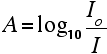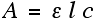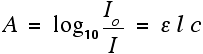THE BEER-LAMBERT LAW

```
```

This page takes a brief look at the Beer-Lambert Law and explains the use of the terms absorbance and molar absorptivity relating to UV-visible absorption spectrometry.

```
```

Absorbance

Measuring the absorbance of a solution

If you have read the page about how an absorption spectrometer works, you will know that it passes a whole series of wavelengths of light through a solution of a substance (the sample cell) and also through an identical container (the reference cell) which only has solvent in it.

Note:  It isn't essential to read about how the spectrometer works, but you could follow this link if you are interested or if it is on your syllabus. Everything you need from that page to understand the present topic is repeated below.

For each wavelength of light passing through the spectrometer, the intensity of the light passing through the reference cell is measured. This is usually referred to as Io - that's I for Intensity.

The intensity of the light passing through the sample cell is also measured for that wavelength - given the symbol, I.

If I is less than Io, then obviously the sample has absorbed some of the light. A simple bit of maths is then done in the computer to convert this into something called the absorbance of the sample - given the symbol, A.

For reasons to do with the form of the Beer-Lambert Law (below), the relationship between A (the absorbance) and the two intensities is given by:On most of the diagrams you will come across, the absorbance ranges from 0 to 1, but it can go higher than that.

An absorbance of 0 at some wavelength means that no light of that particular wavelength has been absorbed. The intensities of the sample and reference beam are both the same, so the ratio Io/I is 1. Log10 of 1 is zero.

An absorbance of 1 happens when 90% of the light at that wavelength has been absorbed - which means that the intensity is 10% of what it would otherwise be.

In that case, Io/I is 100/I0 (=10) and log10 of 10 is 1.

Note:  If you don't feel comfortable with logarithms, don't worry about it. Just accept that an absorbance scale often runs from zero to 1, but could go higher than that in extreme cases (in other words where more than 90% of a wavelength of light is absorbed).

Absorbance isn't very good for making comparisons

The importance of concentration

The proportion of the light absorbed will depend on how many molecules it interacts with. Suppose you have got a strongly coloured organic dye. If it is in a reasonably concentrated solution, it will have a very high absorbance because there are lots of molecules to interact with the light.

However, in an incredibly dilute solution, it may be very difficult to see that it is coloured at all. The absorbance is going to be very low.

Suppose then that you wanted to compare this dye with a different compound. Unless you took care to make allowance for the concentration, you couldn't make any sensible comparisons about which one absorbed the most light.

The importance of the container shape

Suppose this time that you had a very dilute solution of the dye in a cube-shaped container so that the light travelled 1 cm through it. The absorbance isn't likely to be very high. On the other hand, suppose you passed the light through a tube 100 cm long containing the same solution. More light would be absorbed because it interacts with more molecules.

Again, if you want to draw sensible comparisons between solutions, you have to allow for the length of the solution the light is passing through.

Both concentration and solution length are allowed for in the Beer-Lambert Law.

```
```

The Beer-Lambert Law

What the Law looks like

You will find that various different symbols are given for some of the terms in the equation - particularly for the concentration and the solution length. I'm going to use the obvious form where the concentration of the solution is "c" and the length is "l".

Note:  That's obviously "l" for length. The font I'm using won't distinguish between "l" for length and a capital letter "I" (for Intensity). That problem disappears in the equation below - where it is obvious which is which.```
```

You should recognise the expression on the left of this equation as what we have just defined as the absorbance, A. You might also find the equation written in terms of A:

```
``````
```

That's obviously easier to remember than the first one, but you would still have to learn the equation for absorbance. It might be useful to learn it in the form:

```
``````
```

The Greek letter epsilon in these equations is called the molar absorptivity - or sometimes the molar absorption coefficient.

```
```

Molar absorptivity

If you rearrange the simplest of the equations above to give an expression for epsilon (the molar absorptivity), you get:

```
``````
```

Remember that the absorbance of a solution will vary as the concentration or the size of the container varies. Molar absorptivity compensates for this by dividing by both the concentration and the length of the solution that the light passes through. Essentially, it works out a value for what the absorbance would be under a standard set of conditions - the light travelling 1 cm through a solution of 1 mol dm-3.

That means that you can then make comparisons between one compound and another without having to worry about the concentration or solution length.

Values for molar absorptivity can vary hugely. For example, ethanal has two absorption peaks in its UV-visible spectrum - both in the ultra-violet. One of these corresponds to an electron being promoted from a lone pair on the oxygen into a pi anti-bonding orbital; the other from a pi bonding orbital into a pi anti-bonding orbital.

Note:  These jumps are described in detail on the page explaining the theory of UV-visible spectrometry. Depending on your background knowledge, you may have to read another page first, but there is a link to that on the theory page.

The table gives values for the molar absorptivity of a solution of ethanal in hexane. Notice that there are no units given for absorptivity. That's quite common. If you want them, and assuming the length is in cm and the concentration is mol dm-3, the units are mol-1 dm3 cm-1.

```
```
electron jumpwavelength of maximum absorption (nm)molar absorptivity
lone pair to pi anti-bonding orbital29015
pi bonding to pi anti-bonding orbital18010000
```
```

The ethanal obviously absorbs much more strongly at 180 nm than it does at 290 nm. (Although, in fact, the 180 nm absorption peak is outside the range of most spectrometers.)

You may come across diagrams of absorption spectra plotting absorptivity on the vertical axis rather than absorbance. However, if you look at the figures above and the scales that are going to be involved, you aren't really going to be able to spot the absorption at 290 nm. It will be a tiny little peak compared to the one at 180 nm.

To get around this, you may also come across diagrams in which the vertical axis is plotted as log10(molar absorptivity).

If you take the logs of the two numbers in the table, 15 becomes 1.18, while 10000 becomes 4. That makes it possible to plot both values easily, but produces strangely squashed-looking spectra!

Note:  Don't worry too much about this for the purposes of UK A level or its equivalents. What you are going to be mainly concerned with is what wavelengths the absorptions peak at. Exactly how you plot the vertical scale doesn't affect this in any way. Whether you are looking at a plot involving absorbance, molar absorptivity or log10(molar absorptivity), it doesn't affect the wavelengths absorbed. The peaks and troughs of the spectrum just look a bit different (vertically stretched out or squashed), and the vertical scale will have different units - but the peaks will occur at exactly the same wavelengths.

```
```
 Questions to test your understanding If this is the first set of questions you have done, please read the introductory page before you start. You will need to use the BACK BUTTON on your browser to come back here afterwards. questions on the Beer-Lambert Law answers
```
```

Where would you like to go now?

To the UV-visible spectroscopy menu . . .

To the analysis menu . . .

To Main Menu . . .

```
```

© Jim Clark 2007 (modified May 2016)